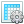## Definition#

Abstraction rules are rules, that initiate the automatical setting of certain answer values by the system, depending on answer values that were provided by the user for previous questions. Values that are to be set can either be derived from other question values or also from complex formulas (see C.).

## Syntax#

### Basic example#

```IF numerical question > 0
THEN other numerical question = 20
```

The above example presents a rule, that assigns the numerical value 20 to the (numerical) question other numerical question in the case that "numerical question" is greater than 0.

```IF numerical question > 130
THEN choice question = increased
```

Also the values of one-choice and multiple-choice questions are set just the same way, as shown right above. There, the one-choice question choice question is set to the value increased.

### Complex expressions#

The value of numeric abstraction questions (both for setting and adding of values, sections A. and B.) can be defined by more complex expressions or formulas.

```IF "Average mileage /100km" > 0 AND "Real mileage  /100km" = known
THEN "Num. Mileage evaluation" = (("Real mileage /100km" / "Average mileage/100km") * 100.0)
```

In the above example, the value for the numerical question Num. Mileage evaluation is defined by a complex formula (which calculates the fraction of the Real mileage per 100km and the Average mileage per 100km and then multiplies the resulting value by 100. Complex expressions like the above also always have to be defined in between brackets, as shown in the example.

In general, complex expressions can be combined from basic elements listed in the subsequent table. Thereby symbols---as S1 and S2---can be the names of numerical questions; in that case, the answer value for that question is used for further calculations. Furthermore, symbols can be simple digits. Finally, symbols are also nestable, which is exemplarily shown in the above example where the expression is a product of a digit and a symbol, that itself is a fraction.

Element Meaning
( S1 + S2 ) adds S1 and S2
( S1 - S2 ) subtracts S2 from S1
( S1 / S2 ) divides S1 by S2
( S1 * S2 ) multiplies S1 and S2
( S1 ) value of S1#### More complex?#

For everything beyond this, there is an additional plugin available. But be careful, this extension is non-LGPL-licensed for commercial use: Extended Expressions: Conditions and actions